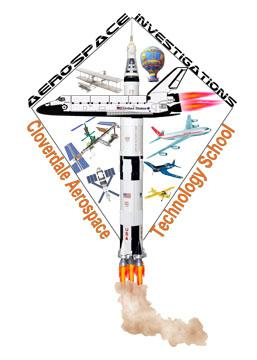# Pre-test, Part 1 Master

24 Questions | Total Attempts: 38SettingsCreate your own QuizINSTRUCTIONS: Answer the questions to the best of your ability. Do not put “IDK” if you do not know an answer, instead answer it to the best of your ability. You will get \$2 or more per question that you answer correctly, \$1 for every question that you try to answer but don’t have the correct answer, and you will get \$0 if you do not try to answer the question or if you put something silly down. These are similar to the questions that you had on your semester pre-test.

• 1.
What does the word "aerodynamics" mean?
• 2.
What are the three states of matter?
• 3.
Essay Question1. **** In the box below, write the word "ESSAY" 2. You will write the actual essay on today's answer document when you get it from Mr. St. Pierre.  You will answer the question on the written document, not on the computer.  This  spot just gives me a place to assign a score once I grade your answer document.
• 4.
Diagram and Label how lift is produced according to Bernoulli's and Newton's theories.1.  In the box below, write the word "LIFT"2. You will complete the diagram on today's answer document when you get it from Mr. St. Pierre.  You will answer the question on the written document, not on the computer.  This  spot just gives me a place to assign a score once I grade your answer document.
• 5.
Bernoulli's Principle states:
• A.

For every action there is an equal and opposite reaction.

• B.

Force equals mass times acceleration.

• C.

As the velocity of a fluid increases, the pressure exerted by the fluid decreases.

• D.

None of the above is correct.

• 6.
The hot air balloon that made the first manned ascent was designed by
• A.

The Montgolfier brothers.

• B.

The Wright brothers.

• C.

Marquis Francois d'Arlandes and Pilatre d'Rozier.

• D.

Chuck Yeager

• 7.
Which engine does not require atmospheric air?
• A.

Reciprocating Engine

• B.

Jet

• C.

V-Inline Engine

• D.

Rocket

• 8.
In this picture, what does the number 27 on the runway represent?
• A.

27° true North

• B.

270° magnetic North

• C.

27° magnetic North

• D.

270° true North

• 9.
Hot air is less dense than cold air, which is how balloons filled with hot air can fly.
• A.

True

• B.

False

• 10.
Looking at the diagram, what action does the aircraft do when it rotates around axis # 1. WORD BANK:  Yaw      Pitch       Roll** Must be spelled correctly.  If not, it will be counted as WRONG.
• 11.
Looking at the diagram, what action does the aircraft do when it rotates around axis # 2. WORD BANK:  Yaw      Pitch       Roll** Must be spelled correctly.  If not, it will be counted as WRONG.
• 12.
Looking at the diagram, what action does the aircraft do when it rotates around axis # 3. WORD BANK:  Yaw      Pitch       Roll** Must be spelled correctly.  If not, it will be counted as WRONG.
• 13.
Of all the wing shapes, the best geometry shape for slow flight is:
• A.

Swept Wing - Low Angle

• B.

Swept Wing - High Angle

• C.

Delta Wing

• D.

Straight wing - Constant Chord

• 14.
This reciprocating engine pictured gets its’ name from what math/geometry principle?
• A.

The RADIUS of a circle

• B.

The DIAMETER of a circle

• C.

The ACUTE ANGLE of a triangle

• D.

The PERIMETER of a square

• 15.
There is breathable oxygen in space.
• A.

True

• B.

False

• 16.
What are the three (3) MAIN types of aerospace engines.  (Not the different kinds of each one)
• A.

Reciprocating

• B.

Turboprop

• C.

• D.

Turbojet

• E.

Scramjet

• F.

V-Inline

• G.

Jet (or Turbine Engine)

• H.

Turbofan

• I.

Plasma Drive

• J.

Rocket

• K.

Horizontally-Opposed

• 17.
Air, while made up of different gasses, has the movement properties of a
• A.

Paper

• B.

Solid

• C.

Fluid

• D.

Organism

• E.

All of the Above

• 18.
Of all the wing shapes, the best geometry shape for fast flight is:
• A.

Swept Wing - Low Angle

• B.

Swept Wing - High Angle

• C.

Delta Wing

• D.

Straight wing - Constant Chord

• 19.
Which of the Four Forces of Flight is an aerodynamic force created by the shape of the airfoil?
• A.

Lift

• B.

Weight

• C.

Thrust

• D.

Drag

• 20.
Which of the Four Forces of Flight is not constant and tries to pull the aircraft to the earth?
• A.

Lift

• B.

Weight

• C.

Thrust

• D.

Drag

• 21.
Which of the Four Forces of Flight pushes / pulls the aircraft through the air?
• A.

Lift

• B.

Weight

• C.

Thrust

• D.

Drag

• 22.
Which of the Four Forces of Flight tries to pull the aircraft backward and slows the aircraft through the air?
• A.

Lift

• B.

Weight

• C.

Thrust

• D.

Drag

Related TopicsBack to top
×

Wait!
Here's an interesting quiz for you.